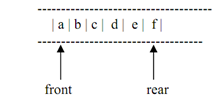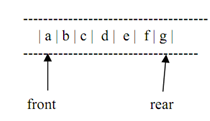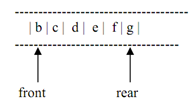## Implementation of queue, Data Structure & Algorithms

Assignment Help:

For a queue a physical analogy is a line at booking counter. At booking counter, customers go to the rear (end) of the line & customers are attended to several services from the front of the line. Unlike stack, customers are inserted at the rear end & deleted from the front end into a queue (FIFO).

In computer science, an instance of the queue is print jobs scheduled for printers. These jobs are maintained into a queue. The job fired for the printer first gets printed first. Similar is the scenario for job scheduling in the CPU of computer.

Like a stack, usually a queue also holds data elements of the similar type. Usually We graphically display a queue horizontally. Figure demonstrates a queue of 5 characters.Figure: A queue of characters

In a queue the rule followed is that elements are inserted at the rear & come off of the front of the queue. After the insertion of an element to the above queue, the position of rear pointer alters as illustrated below. Now the rear is pointing to the new element 'g' added at the rear of the queue.Figure: Queue of above figure after addition of new element

After the elimination of element 'a' from the front, the queue alters to the following along with the front pointer pointing to 'b'.Figure: Queue of figure 5.2 after deletion of an element

Algorithm for insertion of an element to the queue

Step 1: Create a new element to be inserted

Step 2: If the queue is empty, then go to

Step 3, else perform step 4

Step 3: Make the front & rear point this element

Step 4: Insert the element at the end of the queue and shift the rear pointer to the lately added element.

Algorithm for deletion of any element from the queue

Step 1: verify for Queue empty condition. If queue is empty, then go to step 2, else go to step 3

Step 2: Message "Queue Empty"

Step 3: Delete the element through the front of the queue. If in the queue it is the last element, then carry out step a else step b

a) make front & rear point to null

b) Shift the front pointer further on to point to the next element in the queue

#### Explain the halting problem, Explain the halting problem Given a comput...

Explain the halting problem Given a computer program and an input to it, verify whether the program will halt on that input or continue working indefinitely on it.

#### Implementation of stack using arrays, A Stack has an ordered list of elemen...

A Stack has an ordered list of elements & an array is also utilized to store ordered list of elements. Therefore, it would be very simple to manage a stack by using an array. Thoug

#### Define big oh notation, Big oh notation (O) : The upper bound for the funct...

Big oh notation (O) : The upper bound for the function 'f' is given by the big oh notation (O). Considering 'g' to be a function from the non-negative integers to the positive real

#### Recursive function , Q. Write down the recursive function to count the numb...

Q. Write down the recursive function to count the number of the nodes in the binary tree.    A n s . R ecursive Function to count no. of Nodes in Binary Tree is writt

#### Binary search, Explain binary search with an example

Explain binary search with an example

#### Algorithm to delete the specific node from binary searchtree, Q. Write down...

Q. Write down an algorithm to delete the specific node from binary search tree. Trace the algorithm to delete a node (10) from the following given tree. Ans. Algor

#### Graphs, c program to represent a graph as an adjacency multilist form

c program to represent a graph as an adjacency multilist form

#### BINARY SEARCH, GIVE TRACE OF BINARY SEARCH ALGORITHM BY USING A SUITABLE EX...

GIVE TRACE OF BINARY SEARCH ALGORITHM BY USING A SUITABLE EXAMPLE.

#### If a node having two children is deleted from a binary tree, If a node havi...

If a node having two children is deleted from a binary tree, it is replaced by?? Inorder successor